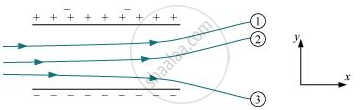Share

# The Figure Shows Tracks of Three Charged Particles in a Uniform Electrostatic Field. Give the Signs of the Three Charges. Which Particle Has the Highest Charge to Mass Ratio? - CBSE (Science) Class 12 - Physics

ConceptElectric Field Electric Field Due to a System of Charges

#### Question

The figure shows tracks of three charged particles in a uniform electrostatic field. Give the signs of the three charges. Which particle has the highest charge to mass ratio?#### Solution

Opposite charges attract each other and same charges repel each other. It can be observed that particles 1 and 2 both move towards the positively charged plate and repel away from the negatively charged plate. Hence, these two particles are negatively charged. It can also be observed that particle 3 moves towards the negatively charged plate and repels away from the positively charged plate. Hence, particle 3 is positively charged.

The charge to mass ratio (emf) is directly proportional to the displacement or amount of deflection for a given velocity. Since the deflection of particle 3 is the maximum, it has the highest charge to mass ratio.

Is there an error in this question or solution?

#### APPEARS IN

NCERT Solution for Physics Textbook for Class 12 (2018 to Current)
Chapter 1: Electric Charge and Fields
Q: 14 | Page no. 47
Solution The Figure Shows Tracks of Three Charged Particles in a Uniform Electrostatic Field. Give the Signs of the Three Charges. Which Particle Has the Highest Charge to Mass Ratio? Concept: Electric Field - Electric Field Due to a System of Charges.
S